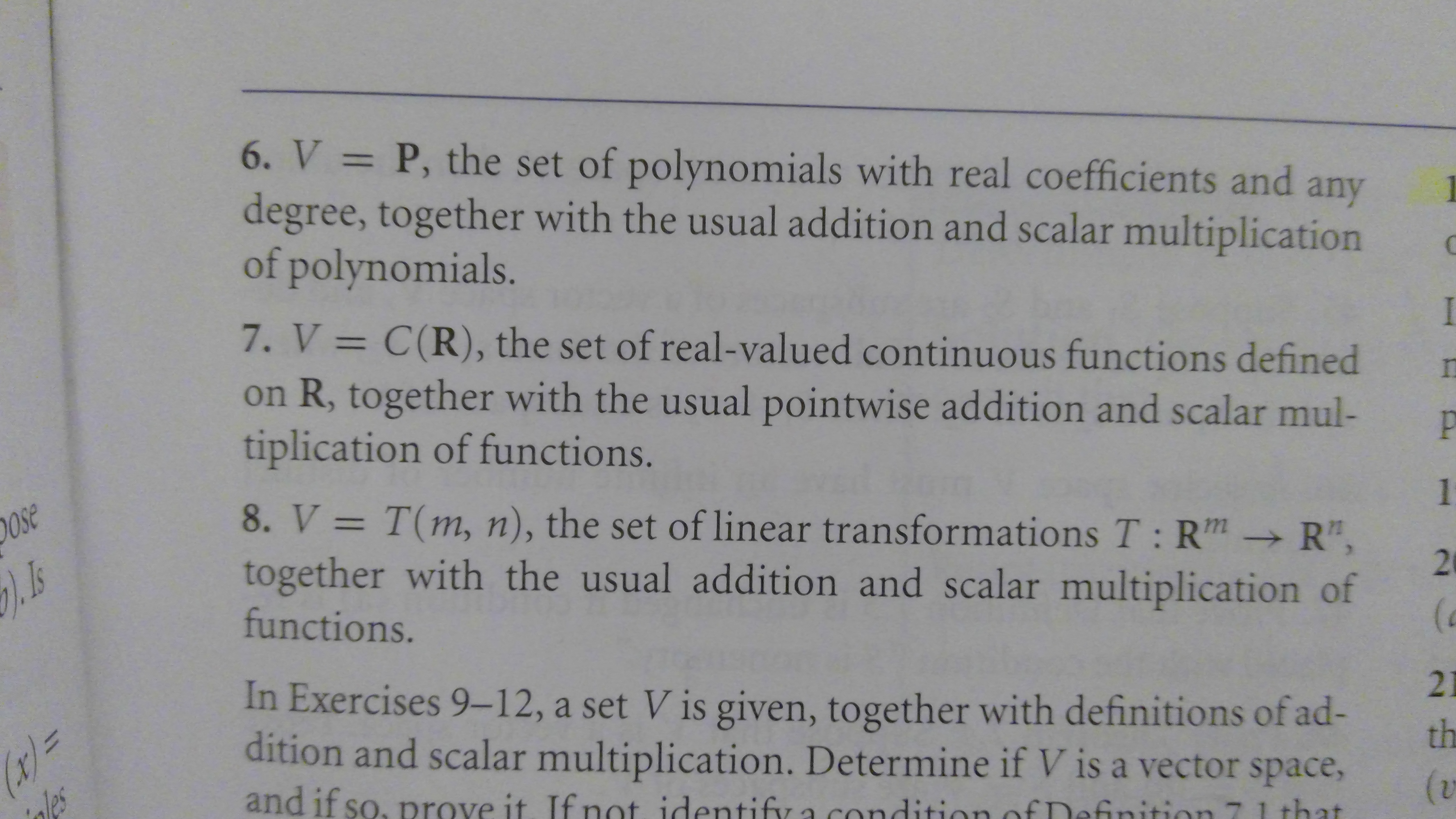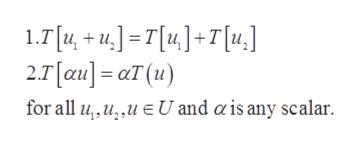# 6. V P, the set of polynomials with real coefficients anddegree, together with the usual addition and scalar multiplicationof polynomials.11any7. V = C(R), the set of real-valued continuous functions definedon R, together with the usual pointwise addition and scalar mul-tiplication of functions.P8. V T(m, n), the set of linear transformations T: Rm R",together with the usual addition and scalar multiplication offunctions.Ose1In Exercises 9-12, a set V is given, together with definitions of ad-dition and scalar multiplication. Determine if V is a vector space,r=nles21thand if so, prove it. If not identify a(vinitionthat29

Question
3 views

How do I verify that Problem #8 is true? I think that is what it is asking for. Also, this question and many other questions in this section are very much conceptual. Although, the math can be used to verify if each question is true. This specific problem is from a linear algebra textbook called  Linear Algebra w/ Applications and the author is by Jeffrey Holt. Right now, I'm in Section 7.1, which consists of what makes a vector space a vector space. Ironically, the author mentioned something new with an R raised to a matrix, as well as a P raised to a number.  Nonetheless, I am most certainly not sure of how to answer the problem, but here are some pictures.help_outlineImage Transcriptionclose6. V P, the set of polynomials with real coefficients and degree, together with the usual addition and scalar multiplication of polynomials. 11 any 7. V = C(R), the set of real-valued continuous functions defined on R, together with the usual pointwise addition and scalar mul- tiplication of functions. P 8. V T(m, n), the set of linear transformations T: Rm R", together with the usual addition and scalar multiplication of functions. Ose 1 In Exercises 9-12, a set V is given, together with definitions of ad- dition and scalar multiplication. Determine if V is a vector space, r= nles 21 th and if so, prove it. If not identify a (v inition that 29 fullscreen
check_circle

Step 1

Given that,

Step 2

Find in the following that V is a vector space.

Since the definition of linear transformation is,

If a function T defined in the following,

Step 3

satisfy the followin...help_outlineImage Transcriptionclose1.T[4, += T +7[4.] 2.7[au aT(u) for all u,,u,,u U and ais any scalar. fullscreen

### Want to see the full answer?

See Solution

#### Want to see this answer and more?

Solutions are written by subject experts who are available 24/7. Questions are typically answered within 1 hour.*

See Solution
*Response times may vary by subject and question.
Tagged in

### Math